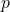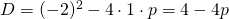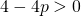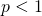# Solution assignment 08 Quadratic equations (abc-formula)

### Assignment 8

For which value(s) ofdoes the equation:have two different solutions.

### Solution

In order to find out whether a quadratic equation has two different solutions, one (or two coinciding) solution or no (real) solution at all, we have to look at the discriminant. In this case we have:If the equation must have two different solutions the discriminant has to satisfy:or:0